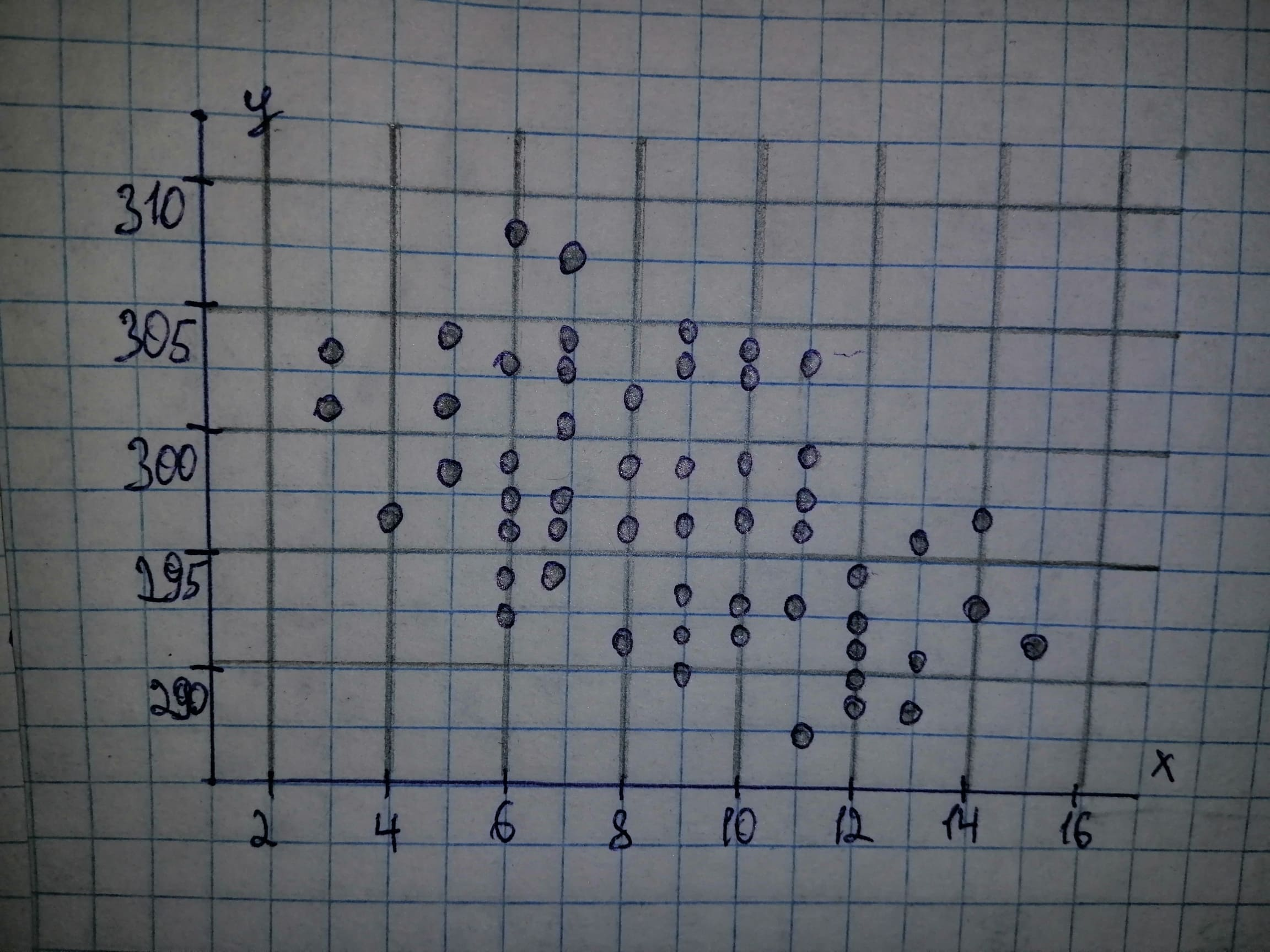Question# How important are birdies (a score of one under par on a given golf hole) in determining the final total score of a woman golfer? From the U.S. Women’

Scatterplots
ANSWEREDHow important are birdies (a score of one under par on a given golf hole) in determining the final total score of a woman golfer? From the U.S. Women’s OpenWeb site, we obtained data on number of birdies during a tournament and final score for 63 women golfers. The data are presented on the WeissStats CD. a) Obtain a scatterplot for the data. b) Decide whether finding a regression line for the data is reasonable. If so, then also do parts (c)-(f). c) Determine and interpret the regression equation for the data. d) Identify potential outliers and influential observations. e) In case a potential outlier is present, remove it and discuss the effect. f) In case a potential influential observation is present, remove it and discuss the effect.2021-02-22

Given: $$\displaystyle{n}=\ \text{Sample size}\ ={63}$$ a) Birdies is on the horizontal axis and Score is on the vertical axis.b) It is reasonable to find a regression lien for the data if there is no strong curvature present in the scatterplot. We note that there is no strong curvature in the scatterplot of part (a) and thus it is reasonable to find a regression line for the data. c) Let us first determine the necessary sums: $$\displaystyle\sum\ {x}_{{{i}}}={570}$$
$$\displaystyle\sum\ {{x}_{{{i}}}^{{{2}}}}={5646}$$
$$\displaystyle\sum\ {y}_{{{i}}}={18717}$$
$$\displaystyle\sum\ {x}_{{{i}}}{y}_{{{i}}}={168943}$$ Next, we can determine $$\displaystyle{S}_{{xx}}$$ and $$\displaystyle{S}_{{{x}{y}}}$$
$$\displaystyle{S}_{{xx}}=\ \sum\ {{x}_{{{i}}}^{{{2}}}}\ -\ {\frac{{{\left(\sum\ {x}_{{{i}}}\right)}^{{{2}}}}}{{{n}}}}={5646}\ -\ {\frac{{{570}^{{{2}}}}}{{{63}}}}={488.8751}$$
$$\displaystyle{S}_{{{x}{y}}}=\ \sum\ {x}_{{{i}}}{y}_{{{i}}}\ -\ {\frac{{{\left(\sum\ {x}_{{{i}}}\right)}{\left(\sum\ {y}_{{{i}}}\right)}}}{{{n}}}}={168943}\ -\ {\frac{{{570}\ \cdot\ {18717}}}{{{63}}}}=\ -{401.2857}$$ The estimate b of the slope $$\displaystyle\beta$$ is the ratio of $$\displaystyle{S}_{{{x}{y}}}$$ and $$\displaystyle{S}_{{xx}}$$: $$\displaystyle{b}=\ {\frac{{{S}_{{{x}{y}}}}}{{{S}_{{xx}}}}}=\ {\frac{{-{401.2857}}}{{{488.8751}}}}=\ -{0.8209}$$ The mean is the sum of all values divided by the number of values: $$\displaystyle\overline{{{x}}}=\ {\frac{{\sum\ {x}_{{{i}}}}}{{{n}}}}=\ {\frac{{{570}}}{{{63}}}}={9.0476}$$
$$\displaystyle\overline{{{y}}}=\ {\frac{{\sum\ {y}_{{{i}}}}}{{{n}}}}=\ {\frac{{{18717}}}{{{63}}}}={297.0952}$$ The estimate a of the intercept $$\displaystyle\alpha$$ is the average of y decreased by the product of the estimate of the slope and the average of x. $$\displaystyle{a}=\ \overline{{{y}}}\ -\ {b}\ \overline{{{x}}}={297.0952}\ -\ {\left(-{0.8209}\right)}\ \cdot\ {9.0476}={304.5221}$$ General least-squares equation: $$\displaystyle\hat{{{y}}}=\ \alpha\ +\ \beta\ {x}$$. Replace $$\displaystyle\alpha$$ by $$\displaystyle{a}={304.5221}$$ and $$\displaystyle\beta$$ by $$\displaystyle{b}=\ -{0.8209}$$ in the general least-squares equation: $$\displaystyle{y}={a}\ +\ {b}{x}={304.5221}\ +\ {\left(-{0.8209}\right)}{x}$$ d) There appear to be no outliers, because no points in the graph deviate strongly from the general pattern in the other points. There appear to be no influential observatioons, because all data values lie near the regression line. e) Not applicable, because we concluded that there are no potential outliers in part (d). f) Not applicable, because we concluded that there are no potential outliers in part (d).